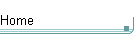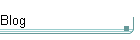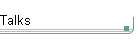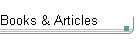GotW #6On theblogNovember 4: Other Concurrency Sessions at PDC November 3 October 26: Hoare on Testing October 23This is the original GotW problem and solution substantially as posted to Usenet. See the book Exceptional C++ (Addison-Wesley, 2000) for the most current solutions to GotW issues #1-30. The solutions in the book have been revised and expanded since their initial appearance in GotW. The book versions also incorporate corrections, new material, and conformance to the final ANSI/ISO C++ standard.

## Const-CorrectnessDifficulty: 6 / 10

Always use const as much as possible, but no more. Here are some obvious and not-so-obvious places where const should be used -- or shouldn't.### Problem

Const is a powerful tool for writing safer code, and it can help compiler optimizations. You should use it as much as possible... but what does "as much as possible" really mean?

Don't comment on or change the structure of this program; it's contrived and condensed for illustration only. Just add or remove "const" (including minor variants and related keywords) wherever appropriate. Bonus Question: In what places are the program's results undefined/uncompilable due to const errors?

```    class Polygon {
public:
Polygon() : area_(-1) {}

void AddPoint( const Point pt ) {
InvalidateArea();
points_.push_back(pt);
}

Point GetPoint( const int i ) {
return points_[i];
}

int GetNumPoints() {
return points_.size();
}

double GetArea() {
if( area_ < 0 ) // if not yet calculated and cached
CalcArea();     // calculate now
return area_;
}

private:
void InvalidateArea() { area_ = -1; }

void CalcArea() {
area_ = 0;
vector<Point>::iterator i;
for( i = points_.begin(); i != points_.end(); ++i )
area_ += /* some work */;
}

vector<Point> points_;
double        area_;
};

Polygon operator+( Polygon& lhs, Polygon& rhs ) {
Polygon ret = lhs;
int last = rhs.GetNumPoints();
for( int i = 0; i < last; ++i ) // concatenate
return ret;
}

void f( const Polygon& poly ) {
}

void g( Polygon& const rPoly ) {
}

void h( Polygon* const pPoly ) {
}

int main() {
Polygon poly;
const Polygon cpoly;
f(poly);
f(cpoly);
g(poly);
h(&poly);
}```### Solution

```    class Polygon {
public:
Polygon() : area_(-1) {}

void AddPoint( const Point pt ) {
InvalidateArea();
points_.push_back(pt);
}```

1. Since the point object is passed by value, there is little or no benefit to declaring it const.

```      Point GetPoint( const int i ) {
return points_[i];
}```

2. Same comment about the parameter as above. Normally const pass-by-value is unuseful and misleading at best.

3. This should be a const member function, since it doesn't change the state of the object.

4. (Arguable.) Return-by-value should normally be const for non-builtin return types. This assists client code by making sure the compiler emits an error if the caller tries to modify the temporary (for example, "poly.GetPoint(i) = Point(2,2);"... after all, if this was intended to work, GetPoint() should have used return-by-reference and not return-by-value in the first place, and as we will see later it makes sense for GetPoint() to return a const value or a const reference since it should be usable on const Polygon objects in operator+()).

Note: Lakos (pg. 618) argues against returning const value, and notes that it is redundant for builtins anyway (for example, returning "const int"), which he notes may interfere with template instantiation.

Guideline: When using return-by-value for non-builtin return types, prefer returning a const value.

```      int GetNumPoints() {
return points_.size();
}```

5. Again, the function should be const.

(It should not return 'const int' in this case, though, since the int is already an rvalue and to put in 'const' can interfere with template instantiation and is confusing, misleading, and probably fattening.)

```      double GetArea() {
if( area_ < 0 ) // if not yet calculated and cached
CalcArea();     // calculate now
return area_;
}```

6. Even though it modifies the object's internal state, this function should be const because the object's observable state is unchanged (we are doing some caching, but that's an implementation detail and the object is logically const). This means that area_ should be declared mutable. If your compiler doesn't support mutable yet, kludge this with a const_cast of area_ (and a comment to remove the cast when mutable is available!), but do make the function const.

```    private:
void InvalidateArea() { area_ = -1; }```

7. While this one is debatable, I'd still recommend that this function ought also to be const, even if for no other reason than consistency. (Granted, semantically it will only be called from non-const functions, since its purpose is to invalidate the cached area_ when the object's state changes.)

```      void CalcArea() {
area_ = 0;
vector<Point>::iterator i;
for( i = points_.begin(); i != points_.end(); ++i )
area_ += /* some work */;
}```

8. This member function definitely should be const. After all, it will be called from another const member function, namely GetArea().

9. Since the iterator should not change the state of the points_ collection, it ought to be a const_iterator.

```      vector<Point> points_;
double        area_;
};

Polygon operator+( Polygon& lhs, Polygon& rhs ) {```

10. Pass by const references, of course.

11. Again, return-by-value should be const.

```      Polygon ret = lhs;
int last = rhs.GetNumPoints();```

12. Since 'last' should never change, say so with "const int".

```      for( int i = 0; i < last; ++i ) // concatenate

(Another reason why GetPoint() should be a const member function, returning either const value or const reference.)

```      return ret;
}

void f( const Polygon& poly ) {

Bonus: This result is undefined if the referenced object is declared as const (which it is in the case of f(cpoly) below). The parameter isn't really const, so don't declare it as const!

```    }

void g( Polygon& const rPoly ) {
}```

13. This 'const' is useless, since references cannot be changed to refer to a different object anyway.

```    void h( Polygon* const pPoly ) {
}```

14. This 'const' is equally useless, but for a different reason: since you're passing the pointer by value, this makes as little sense as passing a parameter 'const int' above.

(If your answer to the bonus part said something about these functions being uncompilable... sorry, they're quite legal C++. You were probably thinking of putting the 'const' to the left of the & or *, which would have made the function body illegal.)

```    int main() {
Polygon poly;
const Polygon cpoly;
f(poly);```

This is fine.

`      f(cpoly);`

This causes undefined results when f() tries to cast away the constness of and then modify its parameter.

`      g(poly);`

This is fine.

`      h(&poly);`

This is fine.

`    }`

That's it. Here's a corrected version (remember, correcting const only, not other poor style):

```    class Polygon {
public:
Polygon() : area_(-1) {}

void  AddPoint( Point pt )       { InvalidateArea();
points_.push_back(pt); }
const Point GetPoint( int i ) const  { return points_[i]; }
int         GetNumPoints() const { return points_.size(); }

double GetArea() const {
if( area_ < 0 ) // if not yet calculated and cached
CalcArea();     // calculate now
return area_;
}

private:
void InvalidateArea() const { area_ = -1; }

void CalcArea() const {
area_ = 0;
vector<Point>::const_iterator i;
for( i = points_.begin(); i != points_.end(); ++i )
area_ += /* some work */;
}

vector<Point>  points_;
mutable double area_;
};

const Polygon operator+( const Polygon& lhs,
const Polygon& rhs ) {
Polygon ret = lhs;
const int last = rhs.GetNumPoints();
for( int i = 0; i < last; ++i ) // concatenate
return ret;
}

void f( Polygon& poly ) {
}

void g( Polygon& rPoly ) { rPoly.AddPoint( Point(1,1) ); }

void h( Polygon* pPoly ) { pPoly->AddPoint( Point(2,2) ); }

int main() {
Polygon poly;
f(poly);
g(poly);
h(&poly);
}```